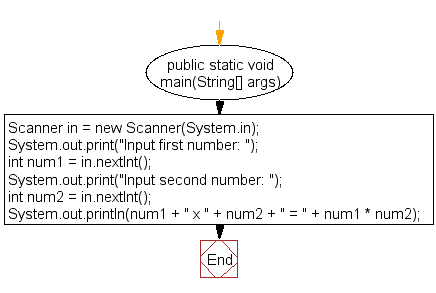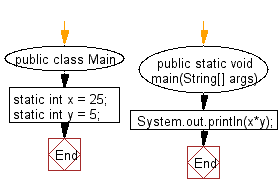﻿ Java exercises: Display the product of two numbers - w3resource# Java Exercises: Display the product of two numbers

## Java Basic: Exercise-5 with Solution

Write a Java program that takes two numbers as input and display the product of two numbers.

Test Data:
Input first number: 25
Input second number: 5

Pictorial Presentation:Sample Solution:

Java Code:

``````import java.util.Scanner;

public class Exercise5 {

public static void main(String[] args) {
Scanner in = new Scanner(System.in);

System.out.print("Input first number: ");
int num1 = in.nextInt();

System.out.print("Input second number: ");
int num2 = in.nextInt();

System.out.println(num1 + " x " + num2 + " = " + num1 * num2);
}

}
```
```

Sample Output:

```Input first number: 25
Input second number: 5
25 x 5 = 125
```

Flowchart:Sample Solution:

Java Code:

``````public class Main
{
static int x = 25;
static int y = 5;
public static void main(String[] args)
{
System.out.println(x*y);
}
}
```
```

Sample Output:

```125
```

Flowchart:Java Code Editor:

What is the difficulty level of this exercise?

Test your Programming skills with w3resource's quiz.

﻿

## Java: Tips of the Day

countOccurrences

Counts the occurrences of a value in an array.

Use Arrays.stream().filter().count() to count total number of values that equals the specified value.

```public static long countOccurrences(int[] numbers, int value) {
return Arrays.stream(numbers)
.filter(number -> number == value)
.count();
}
```

Ref: https://bit.ly/3kCAgLb